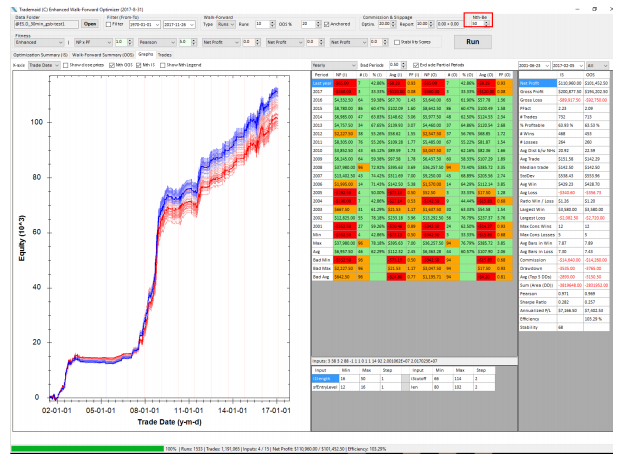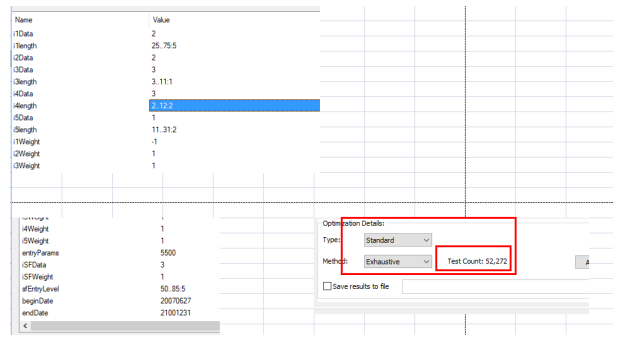## Nth best parameter.

EWFO instead of choosing the best parameter, will chose the nth best.

In the example shown, the 1 to 50th best parameters are shown.

The results are good if the new curves are above and below the original.

If they are strongly below the original curves, this is a fail.TIPS

Typically I like to use 10% of the brute force iterations in a WF as teh amount of iterations in a TS genetic WF.

So it this case below there are 52,272 iterations.

I never keep generations above 100, and I then adjust population so the total number of iterations will be approx 10% of the number used in brute force.

So I would use population of 520, and generations 100.

If this number is too big, it may take too long and or your TS wf files be bigger than you ram. EWFO will not work if you exceed ram size. I have at times used WFO files over 40 GB, but that is rare.

You may also have to increase the step between numbers to reduce the amount of iterations.

Once you have found the range of inputs a system uses, you can always do a second WF with reduced range and reduced step.

ie first run ilength= 15 to 100 step 5

You then see that run ilength only had values to 28 to 50 from optimization.

second run run ilength = 23 to 50 step 5 (or smaller step)If the brute force number of iterations is below 5000, I will just use brute force.